MathScore EduFighter is one of the best math games on the Internet today. You can start playing for free!

## Pythagorean Theorem - Sample Math Practice Problems

The math problems below can be generated by MathScore.com, a math practice program for schools and individual families. References to complexity and mode refer to the overall difficulty of the problems as they appear in the main program. In the main program, all problems are automatically graded and the difficulty adapts dynamically based on performance. Answers to these sample questions appear at the bottom of the page. This page does not grade your responses.

See some of our other supported math practice problems.

### Complexity=3

Find the value of 'x' using the Pythagorean Theorem.

 1.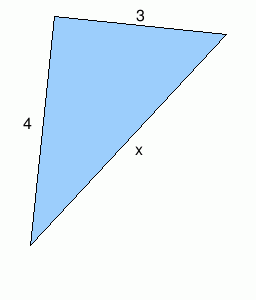x = 2.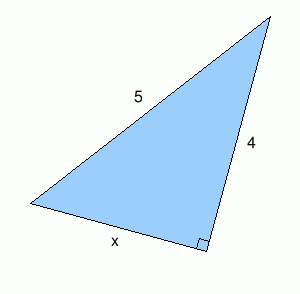x =

### Complexity=5

Find the value of 'x' using the Pythagorean Theorem.

 1.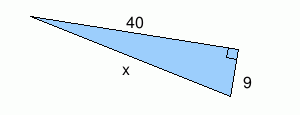x = 2.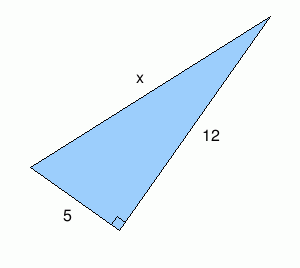x =

### Complexity=8

Find the value of 'x' using the Pythagorean Theorem.

 1.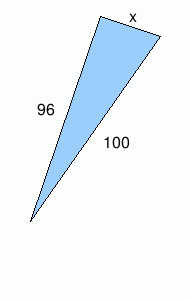x = 2.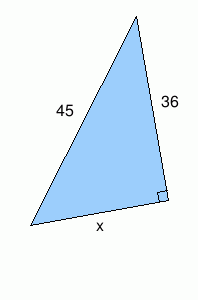x =

### Complexity=3

Find the value of 'x' using the Pythagorean Theorem.

1x =
Solution
a2 + b2 = c2 where c is the hypotenuse (the side opposite the right angle)
c2 = 32 + 42
c2 = 9 + 16
c2 = 25
c = 5
2x =
Solution
a2 + b2 = c2 where c is the hypotenuse (the side opposite the right angle)
a2 = c2 - b2
a2 = 52 - 42
a2 = 25 - 16
a2 = 9
a = 3

### Complexity=5

Find the value of 'x' using the Pythagorean Theorem.

1x =
Solution
a2 + b2 = c2 where c is the hypotenuse (the side opposite the right angle)
c2 = 92 + 402
c2 = 81 + 1600
c2 = 1681
c = 41
2x =
Solution
a2 + b2 = c2 where c is the hypotenuse (the side opposite the right angle)
c2 = 52 + 122
c2 = 25 + 144
c2 = 169
c = 13

### Complexity=8

Find the value of 'x' using the Pythagorean Theorem.

1x =
Solution
a2 + b2 = c2 where c is the hypotenuse (the side opposite the right angle)
a2 = c2 - b2
a2 = 1002 - 962
a2 = 10000 - 9216
a2 = 784
a = 28
2x =
Solution
a2 + b2 = c2 where c is the hypotenuse (the side opposite the right angle)
a2 = c2 - b2
a2 = 452 - 362
a2 = 2025 - 1296
a2 = 729
a = 27